Checkerboard fun

Thinking about test images (13-Jan-2006, 16-Jul-2011, 19-Jul-2011, 19-Jul-2011) recently prompted me to take a look at the Image Processing Toolbox function checkerboard.

I = checkerboard;
imshow(I)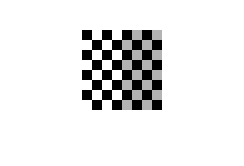The implementation of checkerboard creates a four-by-four set of squares and then uses repmat, something like this:

black = zeros(m);
white = ones(m);
tile = [black white; white black];
I = repmat(tile,p,q);

(Then there's a postprocessing step to turn the white squares into gray squares on the right half of the image.)

This works just fine, but it occurred to me that it's a little inconvenient if you want an image with a size that's not a multiple of the square size. I had an idea for a different implementation of the checkerboard test pattern, one that is based on the function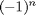where n is an integer. This function bounces back and forth between -1 and 1.

n = 0:10;
(-1).^n
ans =

1    -1     1    -1     1    -1     1    -1     1    -1     1

You can do this in two dimensions, also, with a function like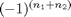.

[n1, n2] = ndgrid(0:4);
(-1).^(n1 + n2)
ans =

1    -1     1    -1     1
-1     1    -1     1    -1
1    -1     1    -1     1
-1     1    -1     1    -1
1    -1     1    -1     1

To hurt your eyes a little, make a bigger version of this and display it as an image.

[n1, n2] = ndgrid(0:199);
I = (-1).^(n1 + n2);
imshow(I, [-1 1])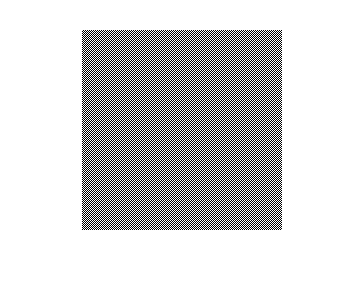This is like a checkerboard but with extra tiny squares. We can make the squares bigger by using some scaling and rounding of the n1 and n2 values.

I = (-1).^(round(n1/20) + round(n2/20));
imshow(I, [-1 1])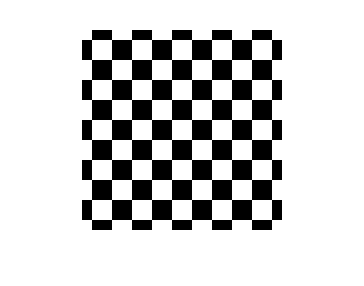Hmm, that resulted in half-tiles around the edges. Let's try floor instead of round.

I = (-1).^(floor(n1/20) + floor(n2/20));
imshow(I, [-1 1])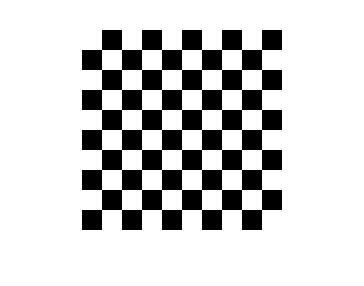Now back to my observation above about the relationship between the size of the image and the number of squares. The implementation based onmakes it very easy to specify independently the image height, image width, and square size. You can even turn the squares into rectangles.

image_height = 206;
image_width = 97;

square_height = 25;
square_width = 11;  % I know, I know; it's not a "square". It's a joke, OK?

[n1, n2] = ndgrid(1:image_height, 1:image_width);
I = (-1).^(floor(n1/square_height) + floor(n2/square_width));
% Make it a binary image of 0s and 1s instead of -1s and 1s.
BW = I == 1;
imshow(BW)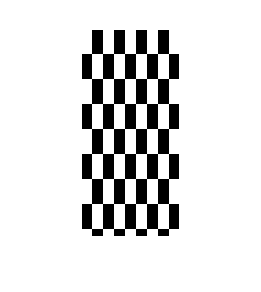Last week's fun with the Jahne test pattern gave me the notion of making a checkboard image with spatially-varying frequency. The technique is the same - square and scale the exponent terms so that the frequency rises as you move away from the center of the image and reaches a maximum at the desired location. (And I'm going to switch back to using round.)

square_size = 20;
[n1, n2] = ndgrid(-100:100);
I = (-1).^(round(n1.^2/1000) + round(n2.^2/1000));
BW = I == 1;
imshow(BW)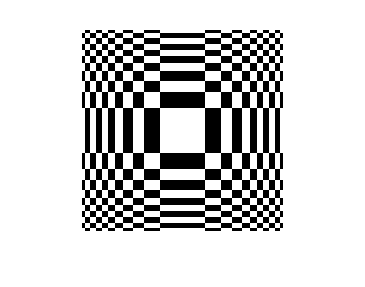I love MATLAB!

Published with MATLAB® 7.12

|